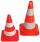# Pythagorean theorem + volume - math problems

#### Number of problems found: 155

• Hexagonal pyramidCalculate the volume and the surface of a regular hexagonal pyramid with a base edge length of 3 cm and a height of 5 cm.
• Tetrahedral pyramidCalculate the regular tetrahedral pyramid's volume and surface if the content area of the base is 20 cm2, and the deviation angle of the side edges from the plane of the base is 60 degrees.
• School modelThe beech school model of a regular quadrilateral pyramid has a base 20 cm long and 24 cm high. Calculate a) the surface of the pyramid in square decimeters, b) the mass of the pyramid in kilograms if the density of the beech is ρ = 0,8 g/cm ^ 3
• Ratio-cuboidThe lengths of the edges of the cuboid are in the ratio 2: 3: 6. Its body diagonal is 14 cm long. Calculate the volume and surface area of the cuboid.
• Cone sideCalculate the volume and area of the cone whose height is 10 cm and the axial section of the cone has an angle of 30 degrees between height and the cone side.
• Traffic conesForty identical traffic cones with a base diameter d = 3 dm and a height v = 6 dm should be painted on the outside with orange paint (without base). How many crowns do we pay for color? If we need 50 cm ^ 3 of paint to paint, 1m2 and 1l of paint costs CZ
• Pine woodFrom a trunk of pine 6m long and 35 cm in diameter with a carved beam with a cross-section in the shape of a square so that the square had the greatest content area. Calculate the length of the sides of a square. Calculate the volume in cubic meters of lu
• Four sided prismCalculate the volume and surface area of a regular quadrangular prism whose height is 28.6cm and the body diagonal forms a 50-degree angle with the base plane.
• Cube in a sphereThe cube is inscribed in a sphere with a volume 7253 cm3. Determine the length of the edges of a cube.
• Hexagon rotationA regular hexagon of side 6 cm is rotated through 60° along a line passing through its longest diagonal. What is the volume of the figure thus generated?
• Triangular prism - regularThe regular triangular prism is 7 cm high. Its base is an equilateral triangle whose height is 3 cm. Calculate the surface and volume of this prism.
• Lateral surface areaThe ratio of the area of the base of the rotary cone to its lateral surface area is 3: 5. Calculate the surface and volume of the cone, if its height v = 4 cm.
• Triangular prismThe triangular prism has a base in the shape of a right triangle, the legs of which is 9 cm and 40 cm long. The height of the prism is 20 cm. What is its volume cm3? And the surface cm2?
• CubesOne cube is an inscribed sphere and the other one described. Calculate the difference of volumes of cubes, if the difference of surfaces in 257 mm2.
• Pyramid 8Calculate the volume and the surface area of a regular quadrangular pyramid with the base side 9 cm and side wall with the base has an angle 75°.
• Triangular prismThe base of the perpendicular triangular prism is a right triangle with a leg length of 5 cm. The content area of the largest sidewall of its surface is 130 cm², and the height of the body is 10 cm. Calculate its volume.
• A concrete pedestalA concrete pedestal has a shape of a right circular cone having a height of 2.5 feet. The diameter of the upper and lower bases are 3 feet and 5 feet, respectively. Determine the lateral surface area, total surface area, and the volume of the pedestal.
• Isosceles + prismCalculate the volume of the perpendicular prism if its height is 17.5 cm and the base is an isosceles triangle with a base length of 5.8 cm and an arm length of 3.7 cm
• Axial sectionAxial section of the cylinder has a diagonal 40 cm. The size of the shell and the base surface are in the ratio 3:2. Calculate the volume and surface area of this cylinder.
• CuboidCuboid with edge a=6 cm and space diagonal u=31 cm has volume V=900 cm3. Calculate the length of the other edges.

Do you have an exciting math question or word problem that you can't solve? Ask a question or post a math problem, and we can try to solve it.

We will send a solution to your e-mail address. Solved examples are also published here. Please enter the e-mail correctly and check whether you don't have a full mailbox.

Tip: Our volume units converter will help you with the conversion of volume units. Pythagorean theorem is the base for the right triangle calculator. Pythagorean theorem - math problems. Volume - math problems.